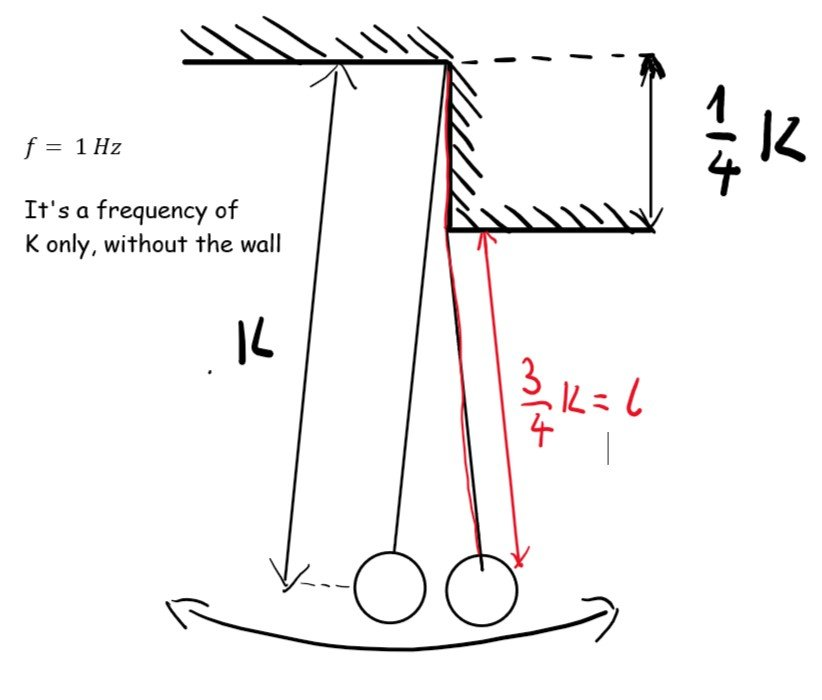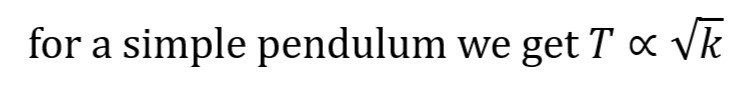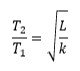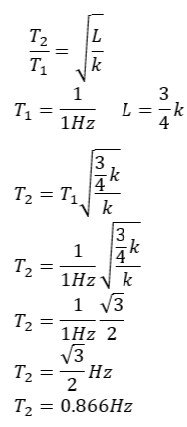# Simple pendulum with a twist

## Recommended Posts

hey It's me again, I need  Help with this pendulum problem,

it is easy
The frequency of vibrations (fluctuations) with a small amplitude of the ball
attached to a weightless string is 1 Hz. I have to find the frequency
of small vibrations of this body suspended on the same string near the edge
shown in the picture. The distance d of the edge from the suspension point is 1/4 k.it's easy but i have trouble with comparing it to the "seconds pendulum"

Because with seconds pendulum we get frequency f= 1/2 Hz.

but here we haveso can we compare the two periods like this?so plugging these in I got this:is this correct?

##### Share on other sites

@studiot I guess I have to yet find the third period that is of the overall system so (0.867+1)/2 = T3   That is 0.93?

##### Share on other sites

As the football coach said

"It is a game of two halves"

So perhaps you should be considering half periods?

You have dashed off a lot of formulae/calculations but what about the Physics?

Can you describe the Physics of what happens?

Remember this is a mechanical system with no dissipative forces so it obeys the principle of conservation of (mechanical) energy.

I suggest you say something like

Let the bob be drawn a small distance sideways to the left and then released.

Then describe what happens at each significant point in the travel and the Physics of why.

I think the significant points are

1) The initial starting point

2) The bottom of the travel as the string becomes vertical.

3) The bottom of the travel as the string passes vertical.

4) The top of the travel as the string reaches its max rightward excursion.

5) The bottom of the travel as the string again arrives at the vertical.

6) The bottom of the travel as the string passes vertical now travelling leftwards.

7) The top of the leftward travel when the string is back at the initial starting point.

## Create an account

Register a new account